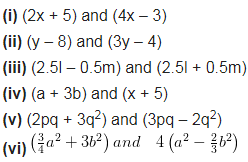# Multiply the binomials :(i) (2x + 5) and (4x – 3)

Multiply the binomials